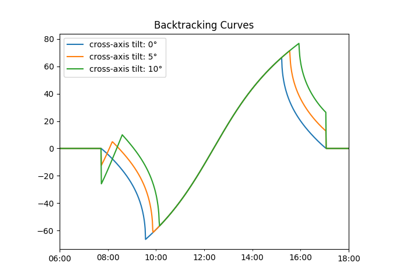# pvlib.tracking.calc_axis_tilt#

pvlib.tracking.calc_axis_tilt(slope_azimuth, slope_tilt, axis_azimuth)[source]#

Calculate tracker axis tilt in the global reference frame when on a sloped plane. Axis tilt is the inclination of the tracker rotation axis with respect to horizontal, ranging from 0 degrees (horizontal axis) to 90 degrees (vertical axis).

Parameters
• slope_azimuth (float) – direction of normal to slope on horizontal [degrees]

• slope_tilt (float) – tilt of normal to slope relative to vertical [degrees]

• axis_azimuth (float) – direction of tracker axes on horizontal [degrees]

Returns

axis_tilt (float) – tilt of tracker [degrees]

Notes

See 1 for derivation of equations.

References

1

Kevin Anderson and Mark Mikofski, “Slope-Aware Backtracking for Single-Axis Trackers”, Technical Report NREL/TP-5K00-76626, July 2020. https://www.nrel.gov/docs/fy20osti/76626.pdf

## Examples using `pvlib.tracking.calc_axis_tilt`#Backtracking on sloped terrain

Backtracking on sloped terrain